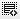What is the difference between Present Value (PV) and Net Present Value (NPV)0
Sir, please explain the answers to both these questions.

What is the difference between Present Value (PV) and Net Present Value (NPV)?

Present value is the current value of a set of cash inflows and outflows and Net present value is the current value of a set of cash inflows - Incorrect

Present value is the current value of a set of cash inflows and outflows and Net present value is the current value of a set of cash outflows

Present value is the current value of a set of cash inflows and Net present value is the current value of a set of cash inflows and outflows - Correct

Present value is the current value of a set of cash inflows and Net present value is the current value of a set of cash outflows

If you invested Rs. 10 lakhs (1 million) in a project and expect to get a steady cash flow of Rs. 1 lakhs (100,000) every year over the next 5 years. What is the NPV of this project assuming prevailing interest rates of 15%?

Rs. 615,067 - Incorrect

Rs.-6,64,784 - Correct

Rs. 500

Rs. 600,0000

The difference between PV and NPV is quite subtle. NPV takes into account the investments (cash inflows) and the returns (cash outflows), while PV takes into account only the cash outflows that the project generates. This is best captured by answer choice 3.
Does that make sense?0

How is the figure of [Rs.-6,64,784 - Correct] arrived at ? I am getting PV of 5 yrs = 370863  and hence NPV = 629137

Click on thisicon to add code snippet.